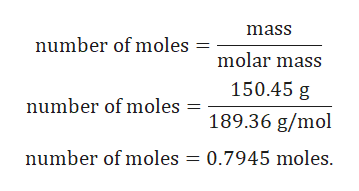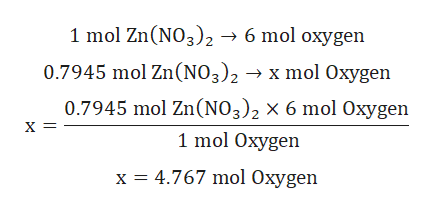Calculate the following quantity: moles of oxygen in 150.45g of Zn(NO3)2

Question

Calculate the following quantity: moles of oxygen in 150.45g of Zn(NO3)2

Step 1

Given:

Mass of Zn(NO3)2 = 150.45 g.

Molar mass of Zn(NO3)2 = 189.36 g/mol.

Step 2

Calculation for number of moles of Zn(NO3)2:help_outlineImage Transcriptionclosemass number of moles molar mass 150.45 g number of moles 189.36 g/mol number of moles = 0.7945 moles fullscreen
Step 3

Calculation for number of moles of Oxygen:

1mole of Zn(NO3...help_outlineImage Transcriptionclose1 mol Zn(NO3)2 6 mol oxygen 0.7945 mol Zn(NO3)2- x mol Oxygen 0.7945 mol Zn(NO3)2 x 6 mol Oxygen X = 1 mol Oxygen 4.767 mol Oxygen x fullscreen

Want to see the full answer?

See Solution

Want to see this answer and more?

Our solutions are written by experts, many with advanced degrees, and available 24/7

See Solution
Tagged in

General Chemistry